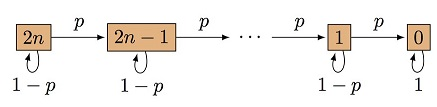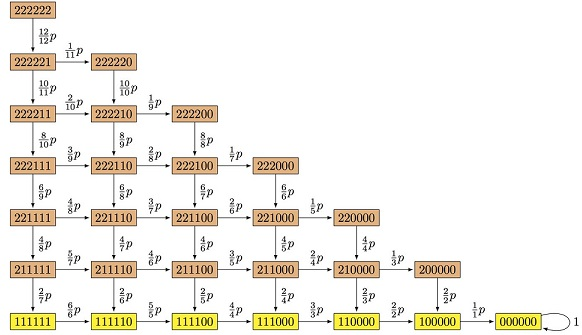# Losing Socks Over a Year

### Problem

#### Sriram Natarajan 1998

This problem was inspired by a April 1997 Scientific American article on Murphy’s law.### Simulation Results

I did a simulation $(100,000$ trials; each trial consists of $365$ days). We begin with $12$ socks $(6$ pairs). If we just multiply $0.019178$ with $365$ we will have an idea of the average number of socks one is expected to lose in any given year. Not surprisingly this number is $7$ (probability was cooked up as $7/365).$ This does not tell us how probable this outcome is or the probability of other possible outcomes (It is possible that one may lose all 12 socks or lose none at all). Below are the simulation results.

$\begin{array}{c|c|cc} \text{Socks}&\text{Number of}&\text{Empirical}&\\ \text{left}&\text{Times}&\text{Probability}&\\ \hline 0&5159&0.05159&&\\ 1&4409& 0.04409&\\ 2&7219& 0.07219&\\ 3&10143& 0.10143&\\ 4&13097& 0.13097&\\ 5&15114& 0.15114&\text{Mode or Most Likely Value}\\ 6&14998& 0.14998&\\ 7&12766& 0.12766&\\ 8&9161& 0.09161&\\ 9&5097& 0.05097&\\ 10&2114& 0.02114&\\ 11&634& 0.00634&\\ 12&89& 0.00089 \end{array}$

The above tells us that we are most likely to lose about $7$ socks $(5$ left). We are extremely unlikely to retain all $12$ socks (less than $1$ in a $1000$ years). How about the number of matching pairs that are left at the end of the year ?

$\begin{array}{c|c|cc} \text{Matching}&\text{Number of}&\text{Empirical}&\\ \text{Pairs Left}&\text{Times}&\text{Probability}&\\ \hline 0&34624&0.34624&\text{Mode or Most Likely Value}\\ 1&29843&0.29843\\ 2&20782&0.20782\\ 3&10283&0.10283\\ 4&3565&0.03565\\ 5&814&0.00814\\ 6&89&0.00089 \end{array}$

Thus there is a $35\%$ chance that all socks remaining do not form a single matching pair. And about a $30\%$ chance that only $1$ single matching pair remains.

### Partial Solution

How does one estimate the probabilities in the first table? Assume we are dealing with 365 days in a year. So on each of those days there is some probability of losing a sock (or not losing one). So immediately the binomial distribution $(0.019178 + 0.980822)^{365}$ looms large. This covers all the $366$ possibilities from losing no socks to losing $365$ socks. However, in this context there are only $12$ socks to lose; so losing any more is not possible and is of no interest.

So we can calculate the probability of losing no socks, that is,

$\displaystyle {365\choose 0}\cdot(0.980822)^{365}$

as well as other possibilities until

$\displaystyle {365\choose 11}\cdot (0.019178)^{11}\cdot (0.980822)^{354}$

(the probability of losing $11$ socks).

The remaining terms can be added to get the probability of losing all 12 socks. More simply we can subtract the sum of the 11 probabilities from $1.0$ to get this value.

$\begin{array}{c|cc} \text{Socks}&\text{Theoretical}&\\ \text{Left}&\text{Probability}&\\ \hline 0&0.051606076\\ 1&0.044849151\\ 2&0.071073130\\ 3&0.102103899\\ 4&0.131644738\\ 5&0.150451786&\text{Mode or Most Likely Value}\\ 6&0.150033356\\ 7&0.127886134\\ 8&0.090588516\\ 9&0.051193102\\ 10&0.021637793\\ 11&0.006080348\\ 12&0.000851966 \end{array}$

That was the easy part. How can one estimate the number of pairs left? Well, supposing we don't lose any socks. Obviously this means we have $6$ intact pairs left. So this scenario has a probability of $0.000851966.$ Suppose we lose $1$ sock. This means we must have 5 intact pairs. However $5$ intact pairs are also possible if we lose $2$ socks. Thus we must calculate the conditional probability of having $5$ intact pairs given the loss of $2$ socks. This is nothing but $\displaystyle {6\choose 5}/{12\choose 10}= 1/11.$ This must be multiplied by $0.021637793$ and summed with $0.006080348$ to get the probability of retaining $5$ pairs. The value $0.008047420$ is very close to the empirical value.

Let $\displaystyle P=\small{p(0)+p(1)+p(2)\cdot\frac{10}{11}+p(3)\cdot\frac{8}{11}+p(4)\cdot\frac{48}{99}+p(5)\cdot\frac{24}{99}+p(6)\cdot\frac{48}{693}}.$

$\begin{array}{c|ll} \text{Matching}&\text{Theoretical}&\\ \text{Pairs Left}&\text{Probability}&\\ \hline 0&0.3460173772164502 =P\\ 1&?????\\ 2&?????\\ 3&?????\\ 4&?????\\ 5&0.008047420 = p(11)+p(10)*1/11\\ 6&0.000851966 = p(12)\\ \end{array}$

### wolframalpha contribution

Following the embedded link we obtain the following:### Wolfram Mathematica### Markov chain

(a) Let $n$ be the initial number of sock pairs, let $p$ be the probability of losing one sock each day, and let $i$ be the number of socks in the drawer; boxes represent the corresponding states. The Markov state graph and corresponding transition matrix are:$M = \left(\begin{array}{cccccc} 1-p & p & 0 & \cdots & 0 & 0 \\ 0 & 1-p & p & \cdots & 0 & 0 \\ \vdots & \vdots & \vdots & \ddots & \vdots & \vdots \\ 0 & 0 & 0 & \cdots & 1-p & p \\ 0 & 0 & 0 & \cdots & 0 & 1 \end{array}\right)$

The vector of probabilities after one year is:

$\mathbf{w} = (1, 0, \ldots, 0) \cdot M^{365}$

and the average number of socks is:

$\bar{s} = \sum_{i=0}^{2n} (2n-i) \, {\bf w}_i$

For $n=6$ and $p=0.019178$, the stochastic vector $\bf w$ is:

$\begin{array}{c|c} i & \mathbf{w}_i\\ \hline 12 & 0.000851966\\ 11 & 0.00608035\\ 10 & 0.0216378\\ 9 & 0.0511931\\ 8 & 0.0905885\\ 7 & 0.127886\\ 6 & 0.150033\\ 5 & 0.150452\\ 4 & 0.131645\\ 3 & 0.102104\\ 2 & 0.0710731\\ 1 & 0.0448492\\ 0 & 0.0516061 \end{array}$

and the average number of socks left in the drawer is:

$\bar{s} = 5.04648 \approx 5.$

For the second part, another Markov chain describes the states of 6 pairs of socks, $s_i \in \{0,1,2\}$ being the occupation numbers of the "color" $i$. Since color is irrelevant for this problem, we may reorder the occupation numbers such that $s_1 \geq s_2 \geq \cdots \geq s_6;$ e.g, the state $222110$ means that the drawer contain $3$ pairs, $2$ unmatched socks, and $1$ missing color. The Markov state graph is:Each node, except for $000000$, is assumed to have an additional loop with probability $q=1-p$, which are hidden for clarity. The states with all socks unmatched are represented in yellow. Reading the state graph lexicographically from top to bottom (and from left to right), the Markov transition matrix $M$ is given below.

The vector of probabilities after one year is:

${\bf w} = (1, 0, \ldots, 0) \cdot M^{365}.$

Finally, the probability $P_0$ of having only unmatched socks after one year is:

$\small{P_0 = {\bf w} \cdot (0,0,0,0,0,0,1,0,0,0,0,0,1,0,0,0,0,1,0,0,0,1,0,0,1,0,1,1)^T \approx 0.346017}.$

In general, the probability $P_k$ of having $k > 0$ matching socks after one year is given by:

\begin{align} P_1 &= \small{{\bf w} \cdot (0,0,0,0,0,1,0,0,0,0,0,1,0,0,0,0,1,0,0,0,1,0,0,1,0,1,0,0)^T \approx 0.29826} \\ P_2 &= \small{{\bf w} \cdot (0,0,0,0,1,0,0,0,0,0,1,0,0,0,0,1,0,0,0,1,0,0,1,0,0,0,0,0)^T \approx 0.206668} \\ P_3 &= \small{{\bf w} \cdot (0,0,0,1,0,0,0,0,0,1,0,0,0,0,1,0,0,0,1,0,0,0,0,0,0,0,0,0)^T \approx 0.103777} \\ P_4 &= \small{{\bf w} \cdot (0,0,1,0,0,0,0,0,1,0,0,0,0,1,0,0,0,0,0,0,0,0,0,0,0,0,0,0)^T \approx 0.0363776} \\ P_5 &= \small{{\bf w} \cdot (0,1,0,0,0,0,0,1,0,0,0,0,0,0,0,0,0,0,0,0,0,0,0,0,0,0,0,0)^T \approx 0.00804742} \\ P_6 &= \small{{\bf w} \cdot (1,0,0,0,0,0,0,0,0,0,0,0,0,0,0,0,0,0,0,0,0,0,0,0,0,0,0,0)^T \approx 0.000851966} \\ \end{align}

### Acknowledgment

Christopher D. Long is responsible for employing wolframalpha to solve the first part. N. N. Taleb used Wolfram's Mathematica for the same purpose. Hélvio Vairinhos solved the problem with Markov chains.